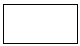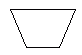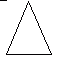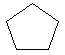Name: ___________________Date:___________________

 Email us to get an instant 20% discount on highly effective K-12 Math & English kwizNET Programs!

### Grade 4 - Mathematics4.9 Polygon Shapes

• Polygons are plane figures with three or more sides.
• A polygon is a closed figure made by joining line segments, where each line segment intersects exactly two others.
• Polygons are named according to the number of sides and angles they have. The most familiar polygons are the triangle, the rectangle, and the square.
• A regular polygon is one that has equal sides.

The table lists all the polygons having up to 10 sides.
 Polygons No. of sides No. of angles No. of vertices No. of diagonals Triangle 3 3 3 0 Quadrilateral 4 4 4 2 Pentagon 5 5 5 5 Hexagon 6 6 6 9 Heptagon 7 7 7 14 Octagon 8 8 8 20 Nonagon 9 9 9 27 Decagon 10 10 10 35

Example: Identify the shapeDirections: Identify the name of the geometric shape for the following questions. Also draw kinds of polygons and write their properties.
 Q 1: What is the name of this shape?trapezoidparallelogramrhombusrectangle Q 2: What is the name of this shape?rectangleparallelogramtrapezoidsquare Q 3: What is the name of this shape?rectangleparallelogramtrapezoidsquare Q 4: What is the name of this shape?trapizoidrectangleparallelogramsquare Q 5: What is the name of this shape?circlesquarerectangletriangle Q 6: What is the name of this shape?octagonhexagonpentagonheptagon Q 7: What is the name of this shape?octagonpentagonhexagonheptagon Q 8: What is the name of this shape?octagonhexagonpentagonheptagon Question 9: This question is available to subscribers only! Question 10: This question is available to subscribers only!